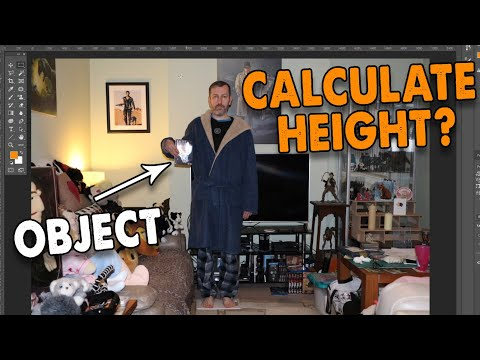# How To Determine Height From A PhotoVideo: Can you Calculate Height from an Object in a Photo? 2023, February

Calculating height from a photograph cannot give a 100% accurate result. But approximate calculations can still be made. You just need to take a close look at the objects that surround the person in the picture. And also it is worth using the observations of anthropologists and trigonometry formulas.

## Instructions

### Step 1

Take a closer look at what is in the photo besides the person. Some items are of constant size. For example, if a person is standing near a standard doorway, car, table, then it is quite possible to imagine his approximate growth.

### Step 2

If there are no landmarks in the photo with which to compare the person being filmed, take a close look at the figure in the photo itself. Anthropologists have noticed that the height of the face of an adult of average height is 1/8 of the body length. If the head is less than this size - for example, 1/9 of the body, then the person in the picture is tall. If the head is large, this is most likely a sign of short stature. This method of calculation can be used only if the person is shot in full height.

### Step 3

Another anthropological method. Measure the width of the shoulders and head of the character in the picture. In a person of average height, the width of the shoulders is equal to twice the width of the head. Also, if the object under study is not completely filmed, you can determine its height by the generally accepted body proportions. For example, the distance from the tip of the hand to the elbow is ¼ of a person's height. The distance from the crown to the nipples is of the same importance. The smaller their ratio, the lower the person.

### Step 4

To determine growth, you can also recall the school curriculum. In particular, the cosine theorem: the square of any side of a triangle (a) is equal to the sum of the squares of the other two sides of the triangle (b and c), minus twice the product of these sides by the cosine of the angle (α) between them. The person whose height you want to know will be in this the case of one of the sides of the triangle. You will find out the length of the remaining two sides in the camera with which the picture was taken. If it is digital, then the distance to the object in it is indicated in the EXIF ​​file. The angle at which the photo was taken can be determined by eye. And then, using the Bradis table, find the cosine value for this angle.